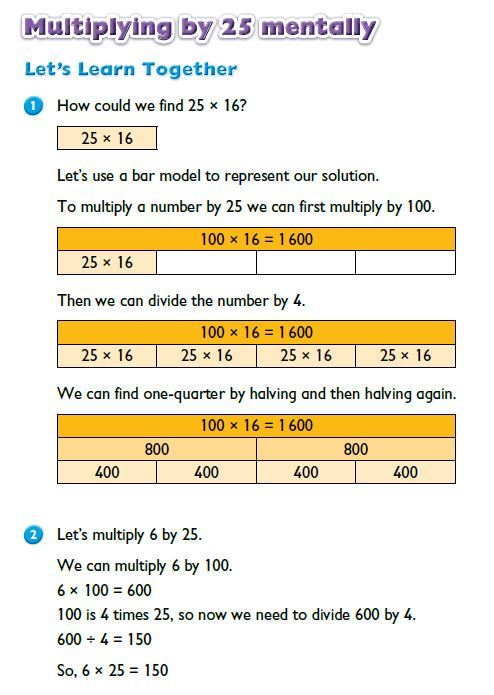## Want to keep learning?

This content is taken from the Macmillan Education's online course, World Class Maths: Asian Teaching Practice. Join the course to learn more.
3.6

## Macmillan Education

Skip to 0 minutes and 0 seconds Multiplying by 25 mentally. An easy strategy to apply, to multiply any number by 25 mentally, is to multiply the number by 100 and then divide by 4. And we can use the bar model to look at how this works. So we know there are four 25s into 100. Rather than multiply 16 by 25, I’m going to multiply 16 by 100. 100 times 16 is 1600. and I’m going to split this into four equal parts. Now, each of these parts should be the equivalent of 25 times 16. So if I divide 1600 by 4 I should be able to find the answer to the question 25 times 16. 1600 divided by 4 is 400. 25 times 16 is 400.

# Explanation: multiplying by 25

This video discusses the following example Bar Model task:Note: These tasks come from ‘Max Maths Primary - A Singapore Approach’.